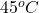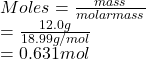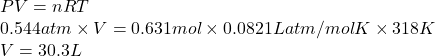## If a sample of 12.0 grams of fluorine gas at 45.00C has a pressure of 0.544 atm, what is the volume of the container? R = 0.082

Question

If a sample of 12.0 grams of fluorine gas at 45.00C has a pressure of 0.544 atm, what is the volume of the container?

R = 0.0821 L atm/mol K

15.1 L

4.29 L

30.3 L

2.14 L

in progress 0
2 months 2021-07-22T15:45:44+00:00 1 Answers 7 views 0

1. Answer: Volume of the container is 30.3 L.

Explanation:

Given: Mass = 12 g

Temperature == (45 + 273) K = 318 K

Pressure = 0.544 atm

R = gas constant = 0.0821 L atm/mol K

Moles is the mass of substance divided by its molar mass.

Hence, moles of fluorine (molar mass = 18.99 g/mol) are as follows.Formula used to calculate the volume of container is as follows.

PV = nRT

where,

P = pressure

V = volume

n = no. of moles

R = gas constant

T = temperature

Substitute the value into above formula as follows.Thus, we can conclude that volume of the container is 30.3 L.